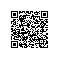1. 创建类
2. 成员方法
3. 构造方法
4. 析构方法
5. 封装
6. 继承
7. 接口
8. 多态
9. 静态成员
10. 常用关键字
11. 常用魔术方法

# 创建类

public $sex; public$age;
}
//实例化ren类
$xiaoli=new ren(); //设置name属性$xiaoli->name="小李";
//获取name属性
echo $xiaoli->name; ?>  输出 =》小李 # 成员方法 就是类中函数，只是在原先的函数声明前面加修饰符public，即可，调用方法$aClass->func()，在函数中使用该对象的属性使用关键字$this，比如$this->name;

class_func.php

<?php
//声明ren类，默认public，不写
class ren{
public $name; public$sex;
public $age; public function say(){ echo "我叫做".$this->name."<br/>";
}
}
//实例化ren类
$xiaoli=new ren(); //设置name属性$xiaoli->name="小李";
//调用方法
$xiaoli->say(); ?>  输出 我叫做小李  # 构造方法 在类的方法中的一个特殊的方法，默认为public（不需要写），，写法：__construct(){}，注意前面是2个下划线。 可以使用构造方法进行对象的初始化赋值 每一次实例化对象（new），都会执行。 construct.php <?php //声明ren类，默认public，不写 class ren{ public$name;
public $sex; public$age;
//成员方法
public function say(){
echo "我叫做".$this->name."<br/>"; echo "性别：".$this->sex."<br/>";
echo "年龄：".$this->age."<br/>"; } //构造方法 function __construct($name,$sex,$age){
$this->name=$name;
$this->sex=$sex;
$this->age=$age;
}
}
//实例化ren类，初始化对象赋值
$xiaoli=new ren("小李","男",25); //调用方法$xiaoli->say();

?>


输出

我叫做小李

# 析构方法

destruct.php

<?php
//声明ren类，默认public，不写
class ren{
public $name; public$sex;
public $age; //成员方法 public function say(){ echo "我叫做".$this->name."<br/>";
echo "性别：".$this->sex."<br/>"; echo "年龄：".$this->age."<br/>";
}
//构造方法
function __construct($name,$sex,$age){$this->name=$name;$this->sex=$sex;$this->age=$age; } //析构方法 function __destruct(){ echo "<br/>$this->name 这个对象被销毁了。<br/>";
}
}
//实例化ren类，初始化对象赋值
$xiaoli=new ren("小李","男",25); //调用方法$xiaoli->say();

//对象自动销毁，执行__destruct

?>


输出

我叫做小李



也可以使用unset方法手动销毁对象，比如$unset($xiaoli)

unset.php

<?php
//声明ren类，默认public，不写
class ren{
public $name; public$sex;
public $age; //成员方法 public function say(){ echo "我叫做".$this->name."<br/>";
echo "性别：".$this->sex."<br/>"; echo "年龄：".$this->age."<br/>";
}
//构造方法
function __construct($name,$sex,$age){$this->name=$name;$this->sex=$sex;$this->age=$age; } //析构方法 function __destruct(){ echo "<br/>$this->name 这个对象被销毁了。<br/>";
}
}
//实例化ren类，初始化对象赋值
$xiaoli=new ren("小李","男",25);$xiaobai=new ren("小白","女",23);
//调用方法
unset($xiaoli,$xiaobai);

//对象自动销毁，执行__destruct

?>


输出

小李 这个对象被销毁了。



# 继承

PHP的面向对象的设计，和Java语言的设计非常的类似，难道是copy“借鉴”了Java的吗？也许吧！

jicheng.php

<?php
//声明tool类
class tool{
function on(){
echo "<br/>启动<br/>";
}
function run(){
echo "运行<br/>";
}
function off(){
echo "关闭<br/>";
}
}
//继承tool类
class fan extends tool{
function app(){
$this->on();$this->run();
$this->off(); } }$fan=new fan();
$fan->app(); ?>  输出 启动 运行 关闭  # 接口 PHP5也是单继承的，只能extends一个类，但是可以实现（相当于继承）多个接口（接口相当于是所有方法都是抽象方法，抽象类） 接口的关键字interface，实现接口implements interface.php <?php //声明接口 interface tool{ //全都是默认的public abstract 的方法 function on(); function run(); function off(); } //实现接口 class fan implements tool{ function on(){ echo "<br/>风扇启动<br/>"; } function run(){ echo "风扇运行<br/>"; } function off(){ echo "风扇关闭<br/>"; } } ?> # 多态 由于PHP是弱类型语言，并不支持完全的多态。 多态就是根据不同的对象来做出不同的反应，运行同一个方法，但是得到的却是不同的结果。 duotai.php <?php //声明接口 interface tool{ //全都是默认的public abstract 的方法 function on(); function run(); function off(); } //实现接口 class fan implements tool{ function on(){ echo "<br/>风扇启动<br/>"; } function run(){ echo "风扇运行<br/>"; } function off(){ echo "风扇关闭<br/>"; } } //实现接口 class engine implements tool{ function on(){ echo "<br/>发动机启动<br/>"; } function run(){ echo "发动机运行<br/>"; } function off(){ echo "发动机关闭<br/>"; } } //状态类 class toolStatus{ function ts($tool){
$tool->on();$tool->run();
$tool->off(); } } //应用多态$fan=new fan();
$engine=new engine();$toolStatus=new toolStatus();
$toolStatus->ts($fan);
$toolStatus->ts($engine);

?>


输出

风扇启动

$fan->run(); ?>  输出 启动 启动 运行 # 常用关键字 final，被final（最终的）修饰的类不能被继承，修饰的属性不能被修改，比如public final function say(){} clone，建立一个对象的副本，比如$newClass=clone $aClass;他会自动调用类的__clone(){}方法 # 常用的魔术方法 function __construct($name,$age){}构造方法 function __destruct(){}析构方法 function __clone(){}拷贝方法 function __set($name,$value)设置方法 function __get($name)获取方法

function __call($func,$values)

function static __callStatic($func,$values)使用钉钉扫一扫加入圈子
+ 订阅